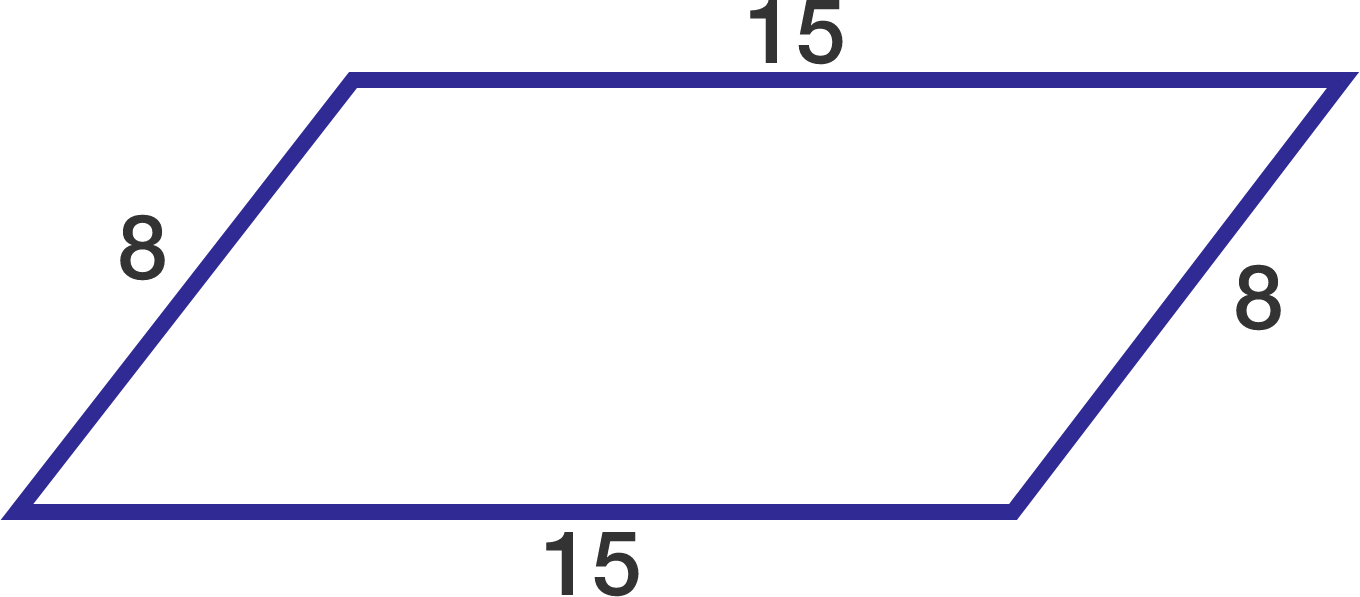# Parallelogram and its diagonal

Geometry Level 2

The lengths of two adjacent sides of a parallelogram are 8 and 15, respectively.

Find the sum of squares of the lengths of the two diagonals.×